Скачать презентацию Crossing the Coexistence Line of the Ising Model

cb5e395dc20a427373498f877a3a4f29.ppt

• Количество слайдов: 29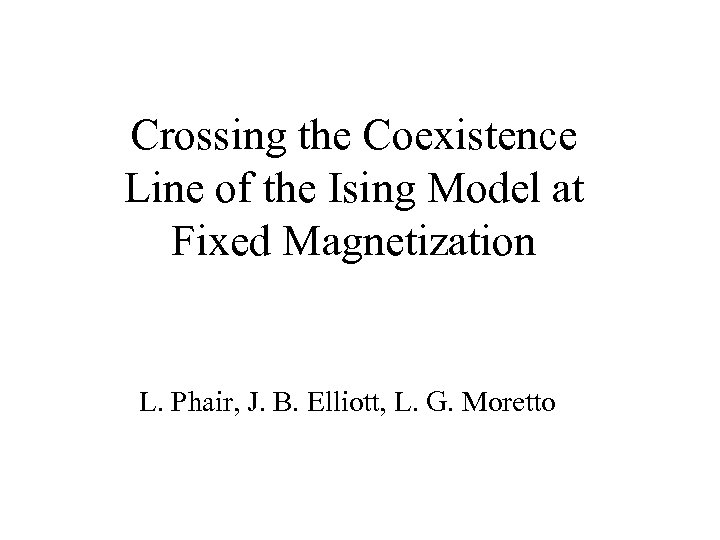Crossing the Coexistence Line of the Ising Model at Fixed Magnetization L. Phair, J. B. Elliott, L. G. Moretto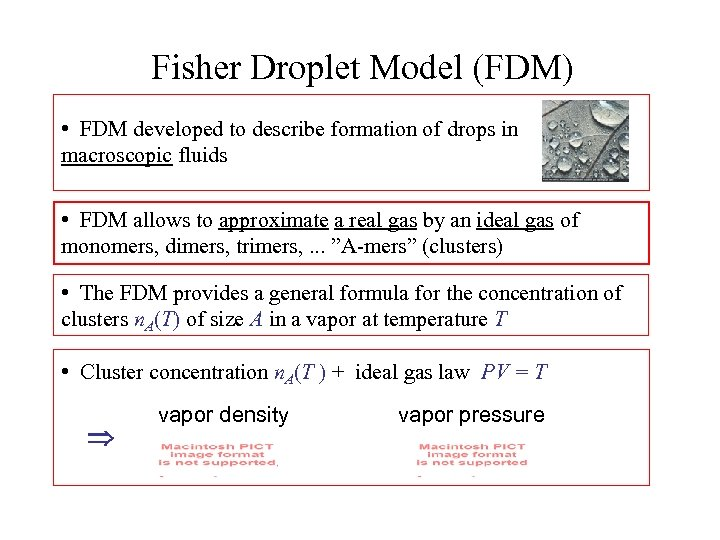Fisher Droplet Model (FDM) • FDM developed to describe formation of drops in macroscopic fluids • FDM allows to approximate a real gas by an ideal gas of monomers, dimers, trimers, . . . ”A-mers” (clusters) • The FDM provides a general formula for the concentration of clusters n. A(T) of size A in a vapor at temperature T • Cluster concentration n. A(T ) + ideal gas law PV = T vapor density vapor pressure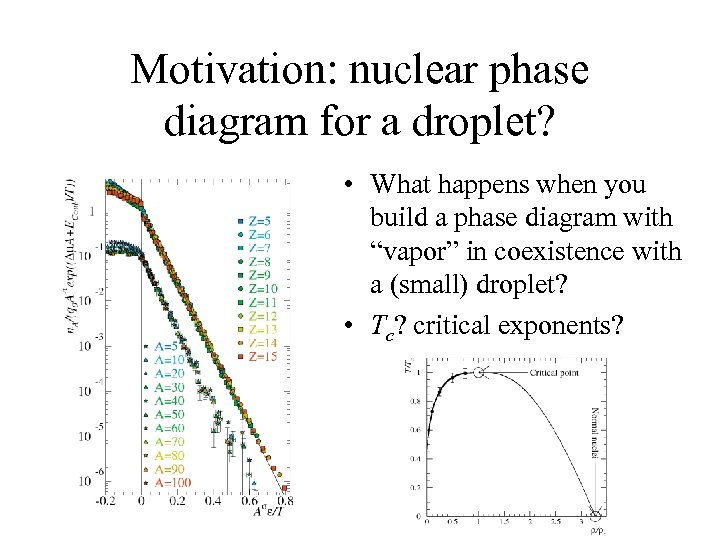Motivation: nuclear phase diagram for a droplet? • What happens when you build a phase diagram with “vapor” in coexistence with a (small) droplet? • Tc? critical exponents?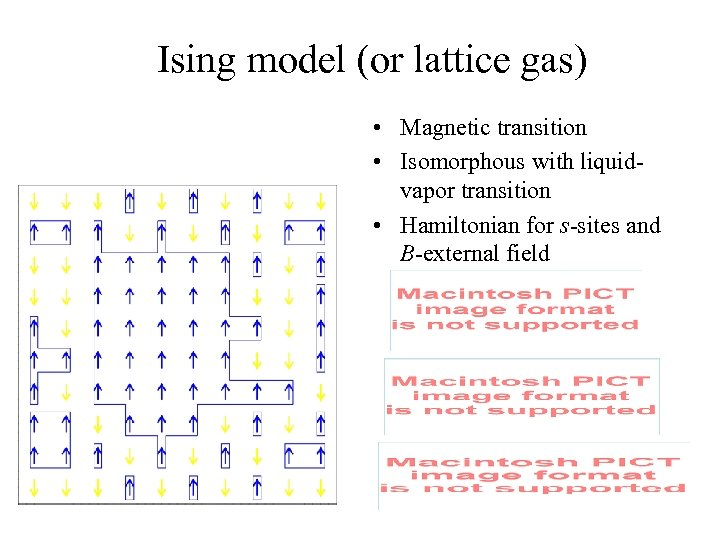Ising model (or lattice gas) • Magnetic transition • Isomorphous with liquidvapor transition • Hamiltonian for s-sites and B-external field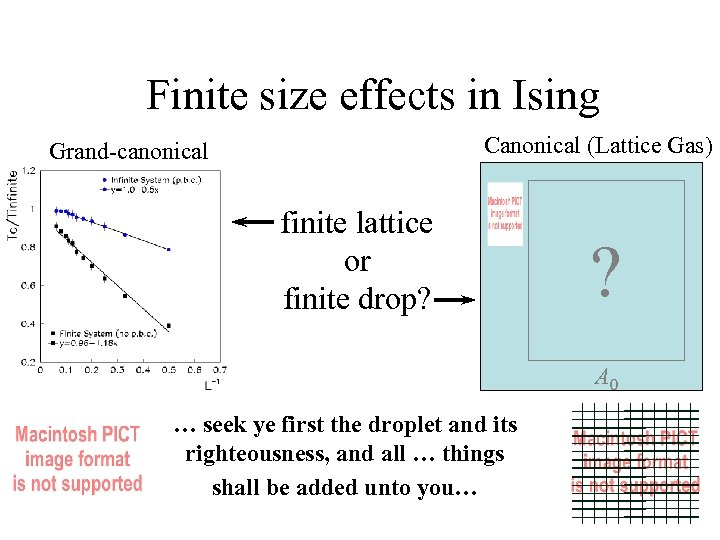Finite size effects in Ising Canonical (Lattice Gas) Grand-canonical finite lattice or finite drop? ? A 0 … seek ye first the droplet and its righteousness, and all … things shall be added unto you…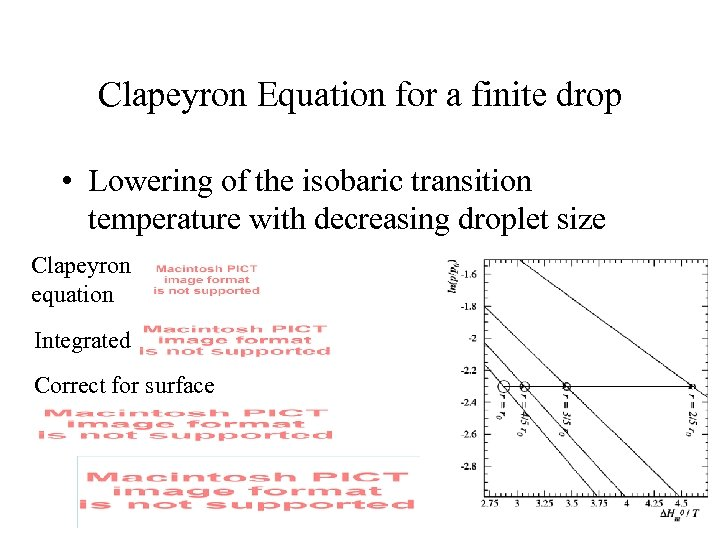Clapeyron Equation for a finite drop • Lowering of the isobaric transition temperature with decreasing droplet size Clapeyron equation Integrated Correct for surface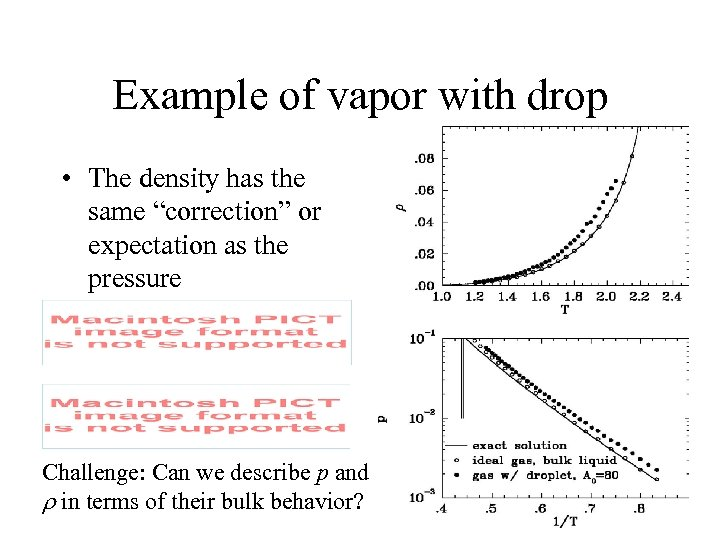Example of vapor with drop • The density has the same “correction” or expectation as the pressure Challenge: Can we describe p and r in terms of their bulk behavior?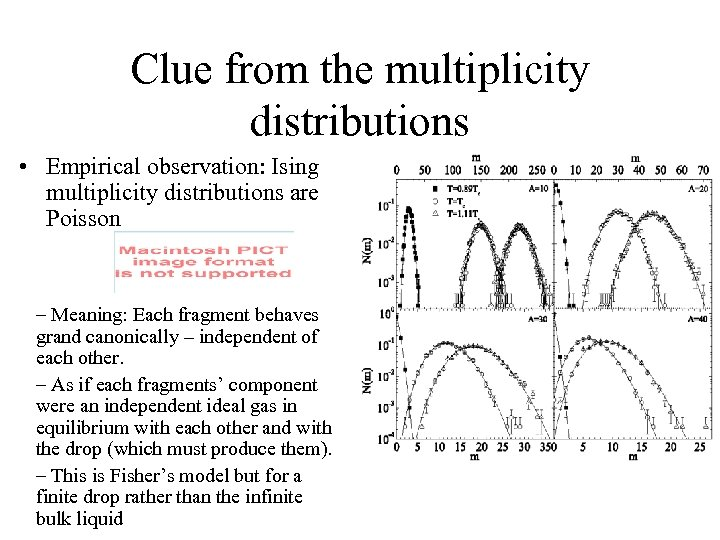Clue from the multiplicity distributions • Empirical observation: Ising multiplicity distributions are Poisson – Meaning: Each fragment behaves grand canonically – independent of each other. – As if each fragments’ component were an independent ideal gas in equilibrium with each other and with the drop (which must produce them). – This is Fisher’s model but for a finite drop rather than the infinite bulk liquid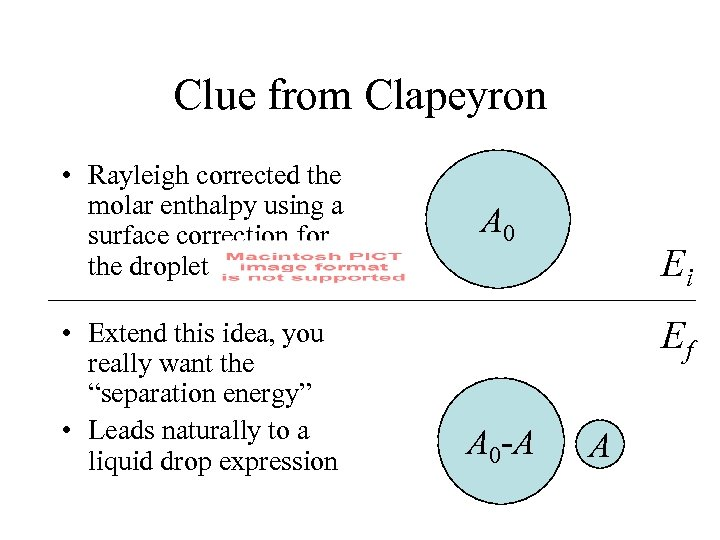Clue from Clapeyron • Rayleigh corrected the molar enthalpy using a surface correction for the droplet • Extend this idea, you really want the “separation energy” • Leads naturally to a liquid drop expression A 0 Ei Ef A 0 -A A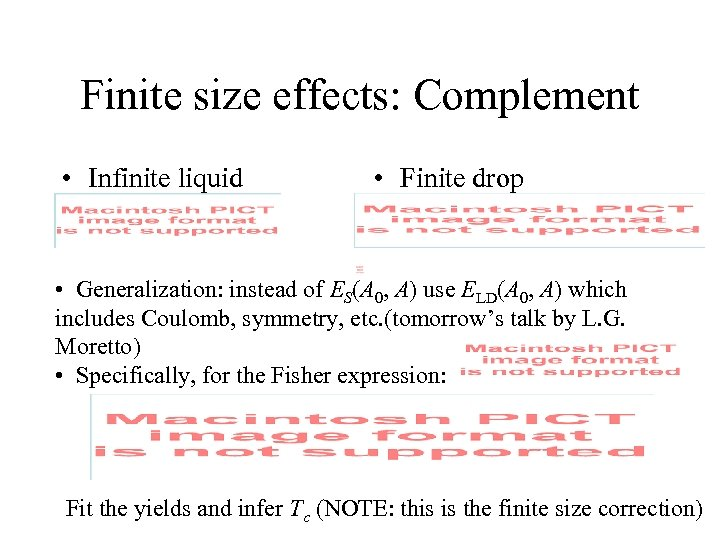Finite size effects: Complement • Infinite liquid • Finite drop • Generalization: instead of ES(A 0, A) use ELD(A 0, A) which includes Coulomb, symmetry, etc. (tomorrow’s talk by L. G. Moretto) • Specifically, for the Fisher expression: Fit the yields and infer Tc (NOTE: this is the finite size correction)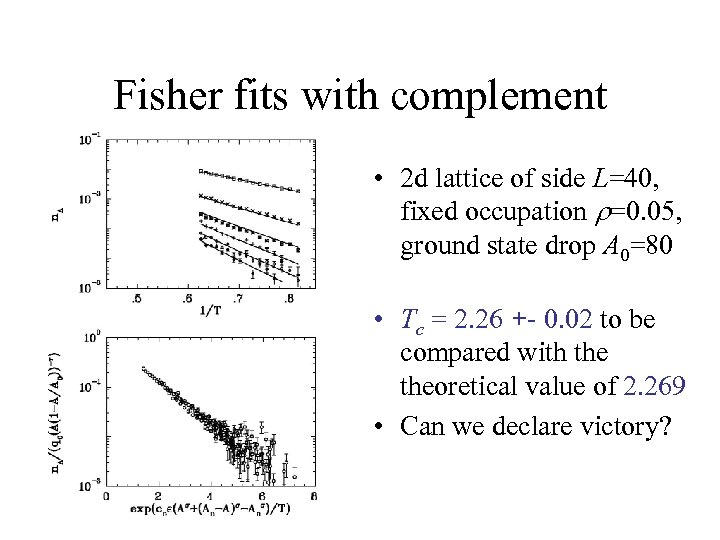Fisher fits with complement • 2 d lattice of side L=40, fixed occupation r=0. 05, ground state drop A 0=80 • Tc = 2. 26 +- 0. 02 to be compared with theoretical value of 2. 269 • Can we declare victory?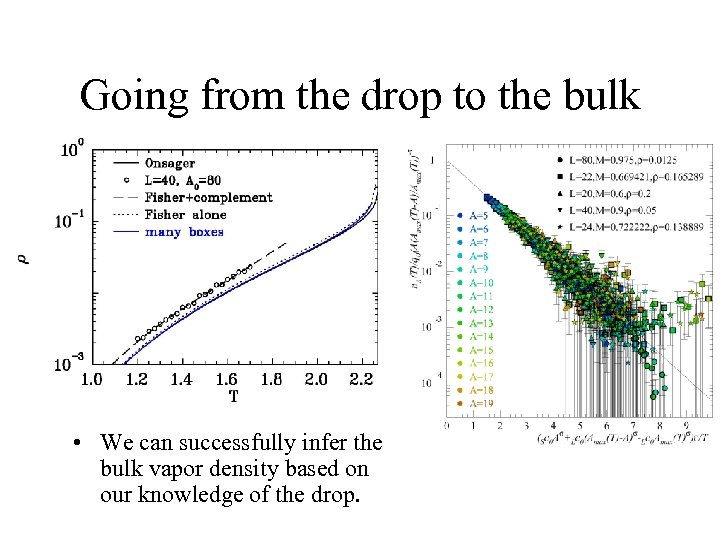Going from the drop to the bulk • We can successfully infer the bulk vapor density based on our knowledge of the drop.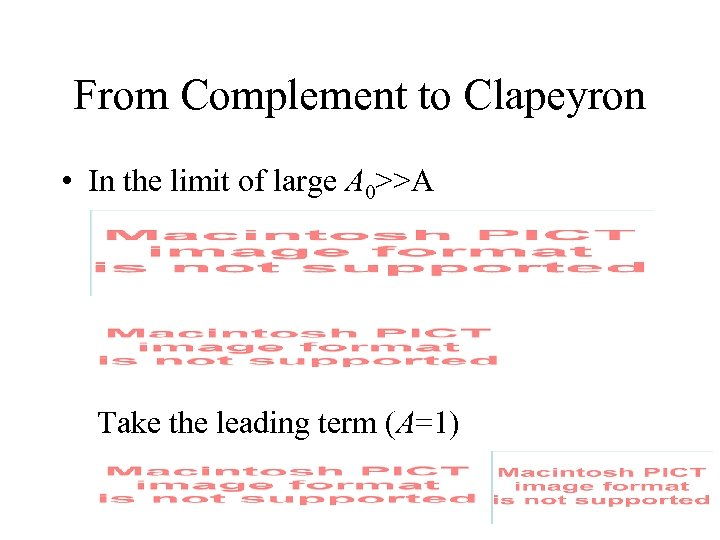From Complement to Clapeyron • In the limit of large A 0>>A Take the leading term (A=1)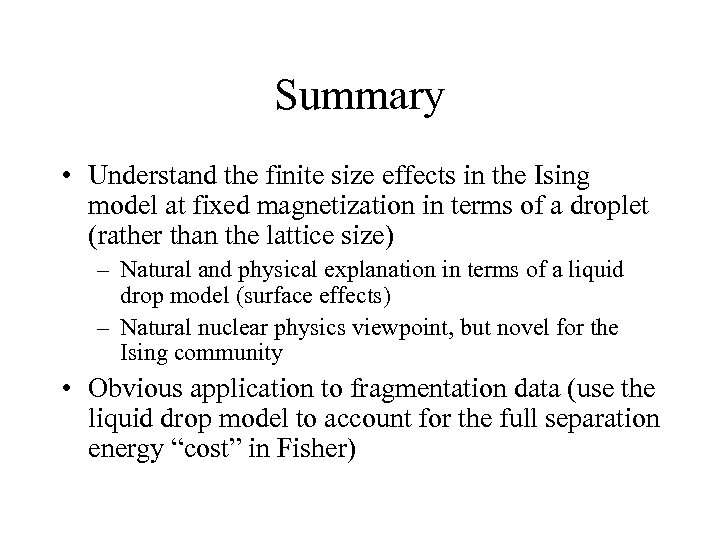Summary • Understand the finite size effects in the Ising model at fixed magnetization in terms of a droplet (rather than the lattice size) – Natural and physical explanation in terms of a liquid drop model (surface effects) – Natural nuclear physics viewpoint, but novel for the Ising community • Obvious application to fragmentation data (use the liquid drop model to account for the full separation energy “cost” in Fisher)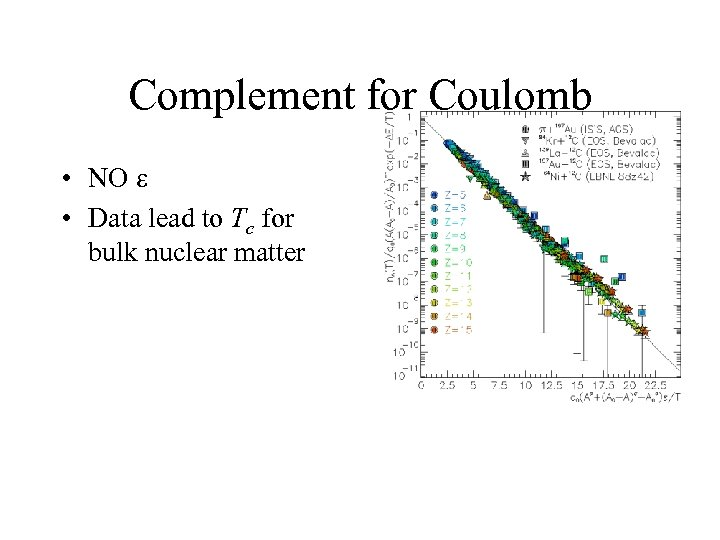Complement for Coulomb • NO e • Data lead to Tc for bulk nuclear matter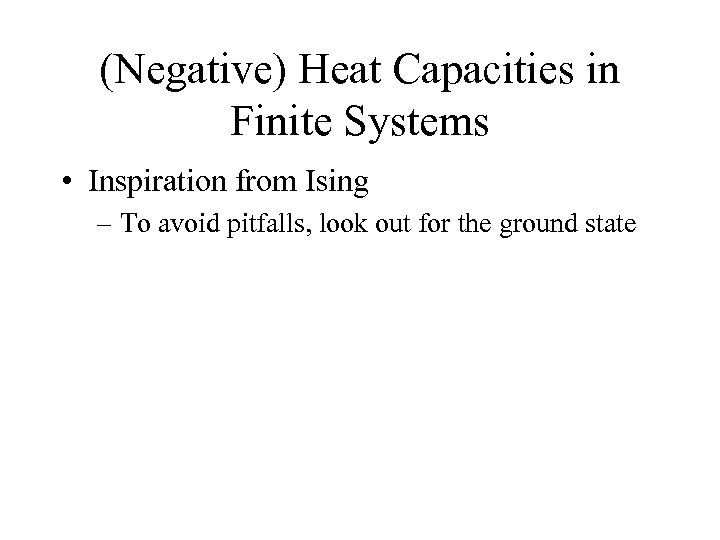(Negative) Heat Capacities in Finite Systems • Inspiration from Ising – To avoid pitfalls, look out for the ground state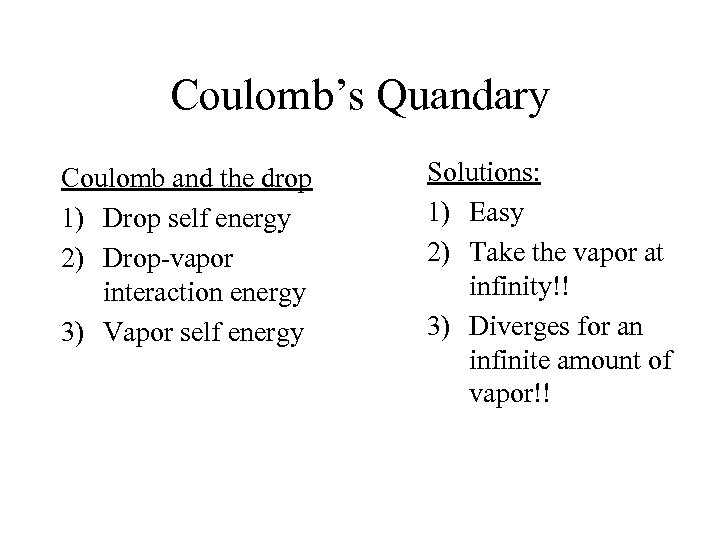Coulomb’s Quandary Coulomb and the drop 1) Drop self energy 2) Drop-vapor interaction energy 3) Vapor self energy Solutions: 1) Easy 2) Take the vapor at infinity!! 3) Diverges for an infinite amount of vapor!!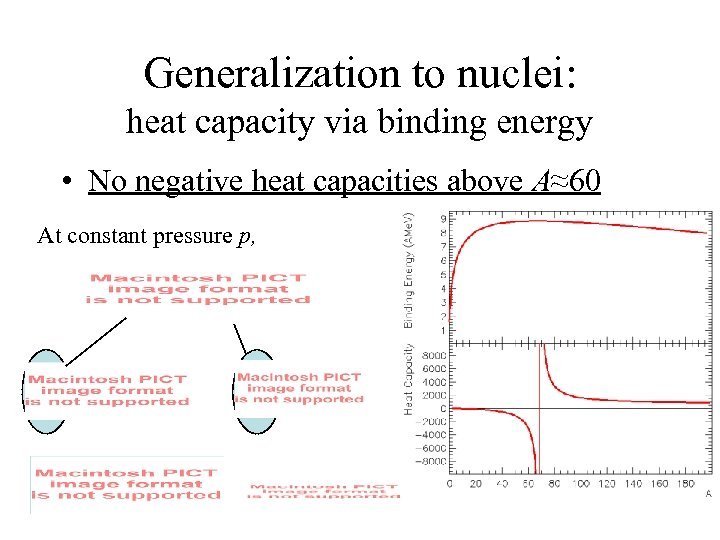Generalization to nuclei: heat capacity via binding energy • No negative heat capacities above A≈60 At constant pressure p,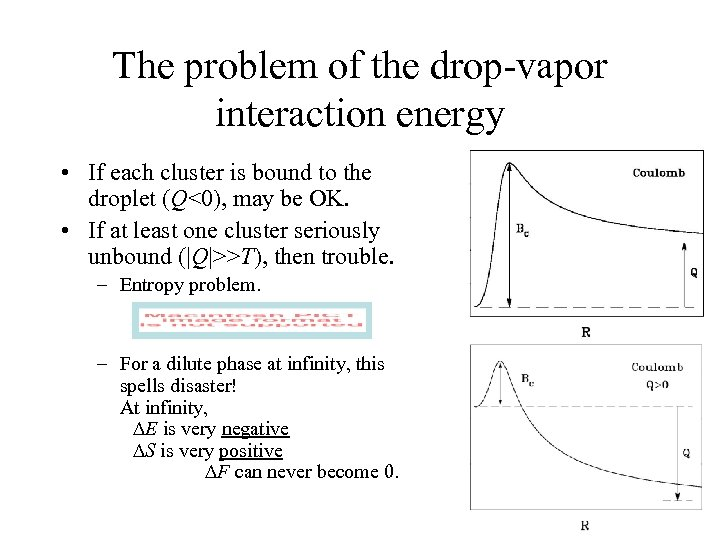The problem of the drop-vapor interaction energy • If each cluster is bound to the droplet (Q<0), may be OK. • If at least one cluster seriously unbound (|Q|>>T), then trouble. – Entropy problem. – For a dilute phase at infinity, this spells disaster! At infinity, DE is very negative DS is very positive DF can never become 0.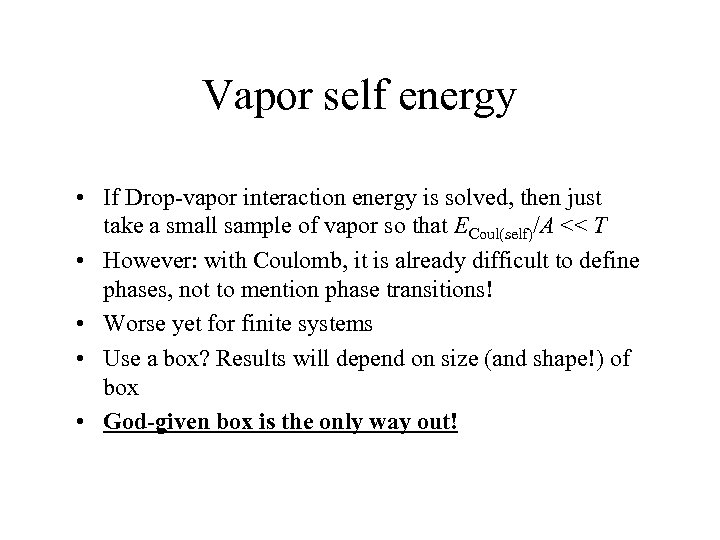Vapor self energy • If Drop-vapor interaction energy is solved, then just take a small sample of vapor so that ECoul(self)/A << T • However: with Coulomb, it is already difficult to define phases, not to mention phase transitions! • Worse yet for finite systems • Use a box? Results will depend on size (and shape!) of box • God-given box is the only way out!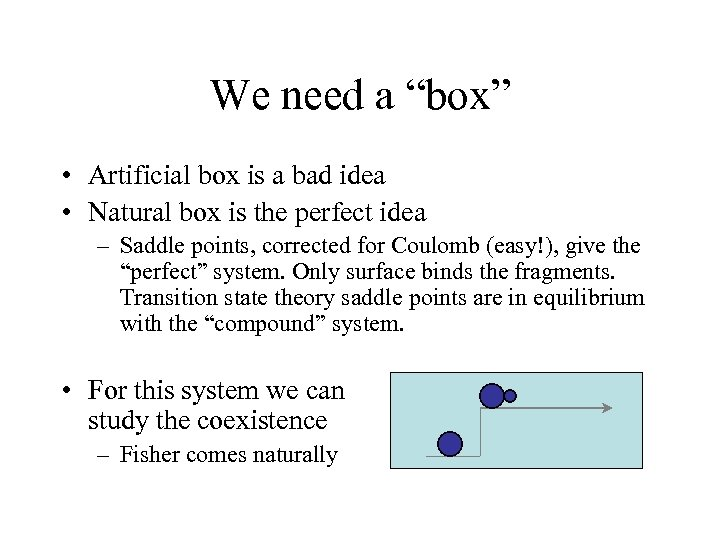We need a “box” • Artificial box is a bad idea • Natural box is the perfect idea – Saddle points, corrected for Coulomb (easy!), give the “perfect” system. Only surface binds the fragments. Transition state theory saddle points are in equilibrium with the “compound” system. • For this system we can study the coexistence – Fisher comes naturally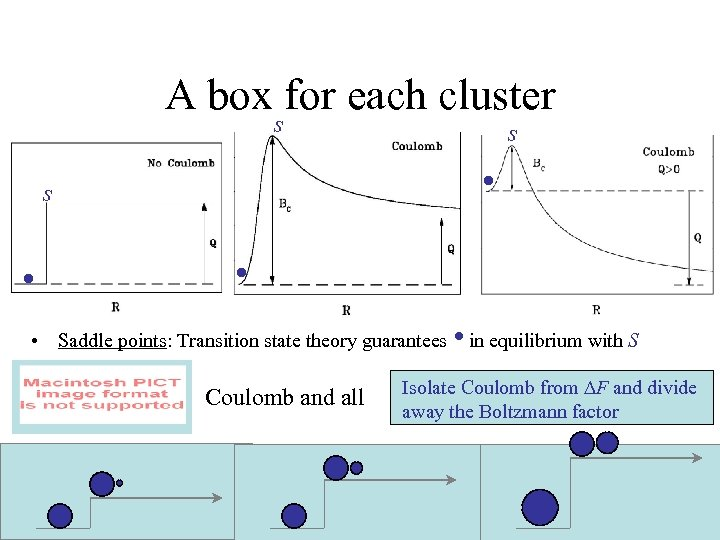A box for each cluster s • • Saddle points: Transition state theory guarantees • in equilibrium with S Coulomb and all Isolate Coulomb from DF and divide away the Boltzmann factor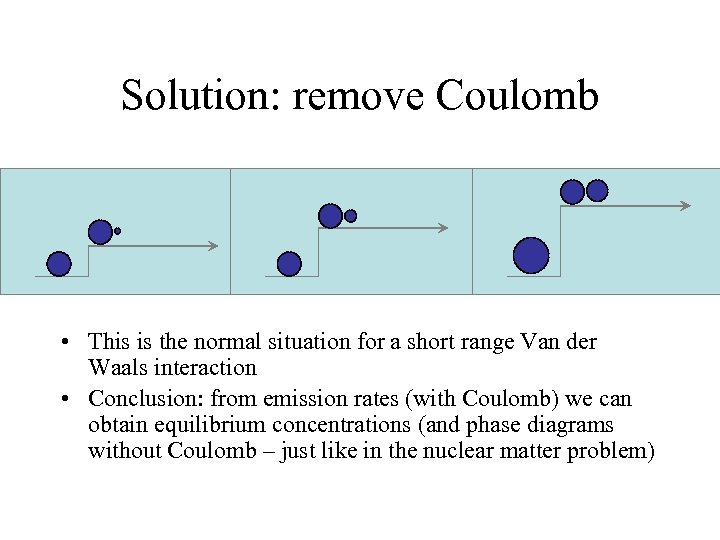Solution: remove Coulomb • This is the normal situation for a short range Van der Waals interaction • Conclusion: from emission rates (with Coulomb) we can obtain equilibrium concentrations (and phase diagrams without Coulomb – just like in the nuclear matter problem)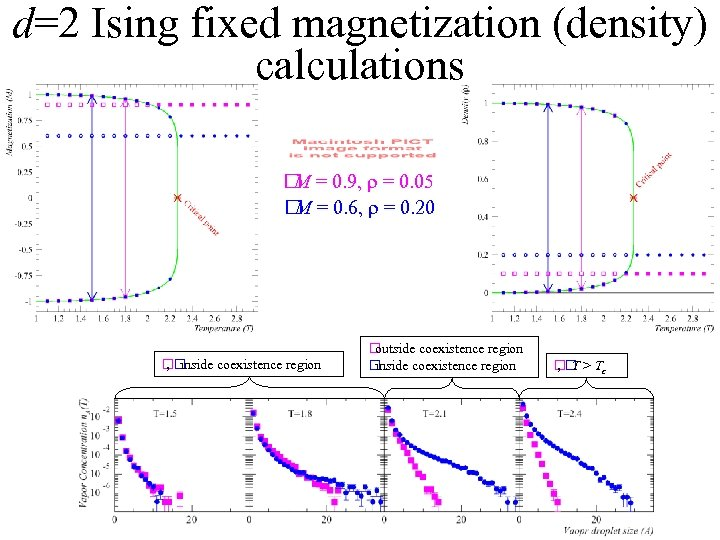d=2 Ising fixed magnetization (density) calculations = 0. 9, r = 0. 05 M = 0. 6, r = 0. 20 M inside coexistence region , outside coexistence region inside coexistence region T > Tc ,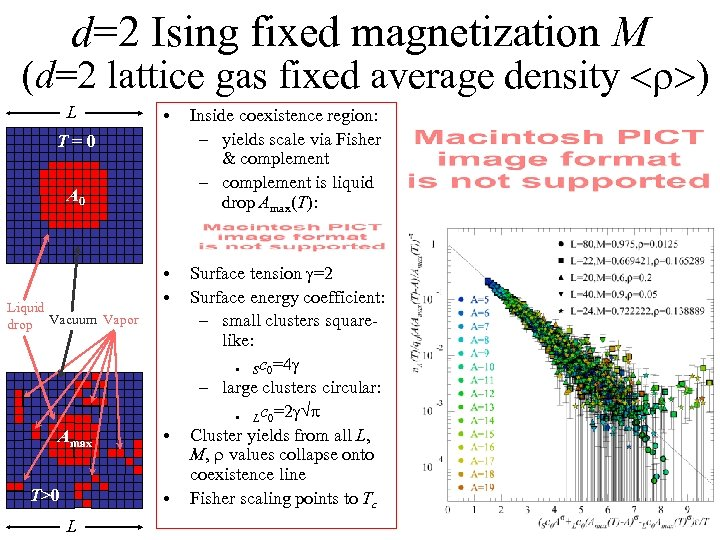d=2 Ising fixed magnetization M (d=2 lattice gas fixed average density ) L • Inside coexistence region: – yields scale via Fisher & complement – complement is liquid drop Amax(T): • • Surface tension g=2 Surface energy coefficient: – small clusters squarelike: • Sc 0=4 g – large clusters circular: • Lc 0=2 g p Cluster yields from all L, M, r values collapse onto coexistence line Fisher scaling points to Tc T=0 A 0 Liquid drop Vacuum Vapor Amax T>0 • • L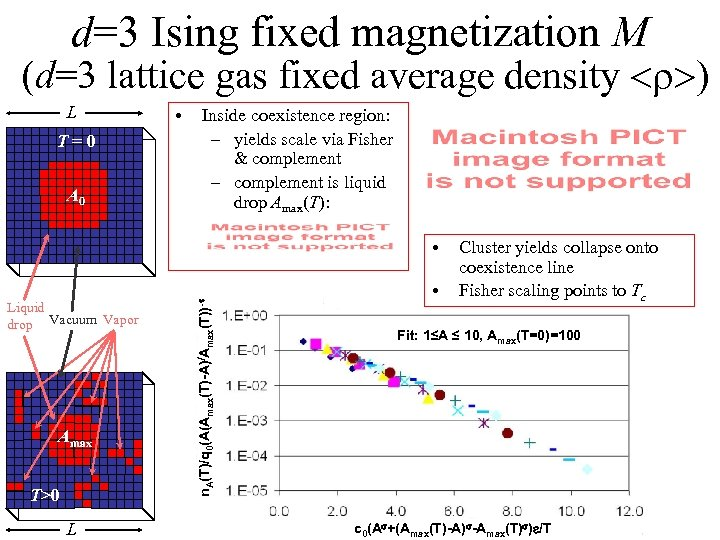d=3 Ising fixed magnetization M (d=3 lattice gas fixed average density ) L T=0 A 0 • Inside coexistence region: – yields scale via Fisher & complement – complement is liquid drop Amax(T): Liquid drop Vacuum Vapor Amax T>0 L n. A(T)/q 0(A(Amax(T)-A)/Amax(T))-t • • Cluster yields collapse onto coexistence line Fisher scaling points to Tc Fit: 1≤A ≤ 10, Amax(T=0)=100 c 0(As+(Amax(T)-A)s-Amax(T)s)e/T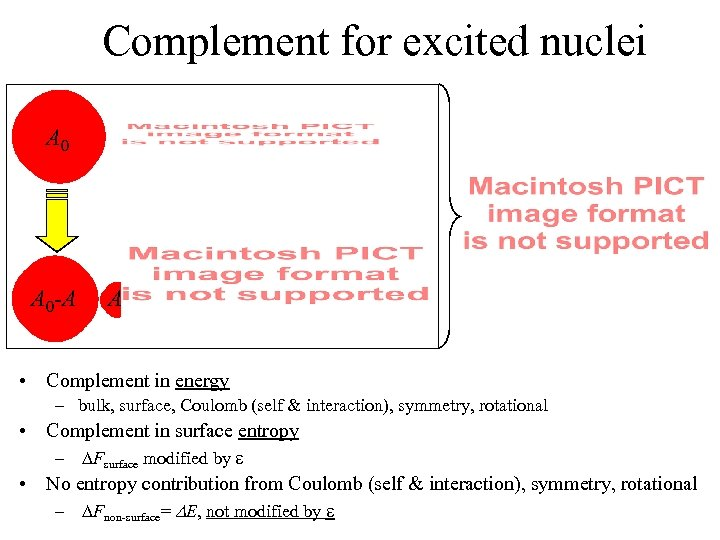Complement for excited nuclei A 0 -A A • Complement in energy – bulk, surface, Coulomb (self & interaction), symmetry, rotational • Complement in surface entropy – DFsurface modified by e • No entropy contribution from Coulomb (self & interaction), symmetry, rotational – DFnon-surface= DE, not modified by e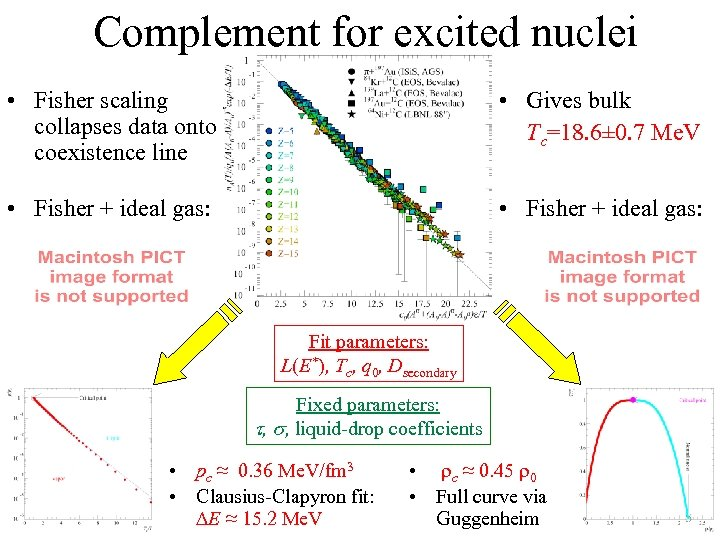Complement for excited nuclei • Fisher scaling collapses data onto coexistence line • Gives bulk Tc=18. 6± 0. 7 Me. V • Fisher + ideal gas: Fit parameters: L(E*), Tc, q 0, Dsecondary Fixed parameters: t, s, liquid-drop coefficients • pc ≈ 0. 36 Me. V/fm 3 • Clausius-Clapyron fit: DE ≈ 15. 2 Me. V • rc ≈ 0. 45 r 0 • Full curve via Guggenheim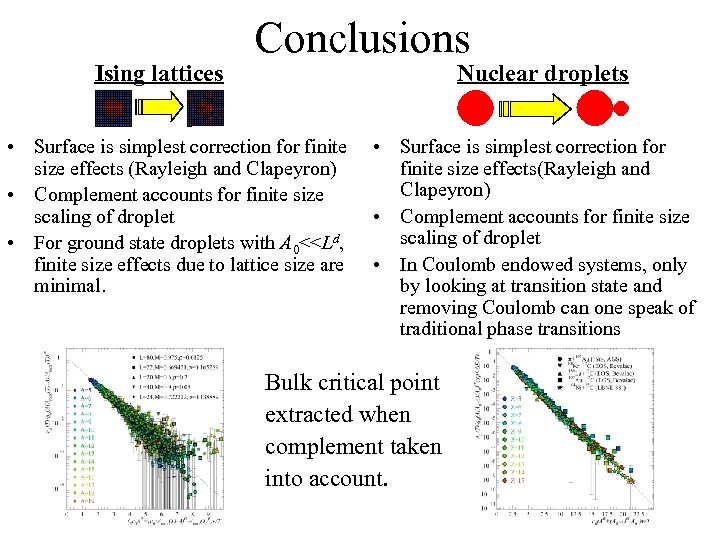Ising lattices Conclusions Nuclear droplets • Surface is simplest correction for finite size effects (Rayleigh and Clapeyron) • Complement accounts for finite size scaling of droplet • For ground state droplets with A 0<